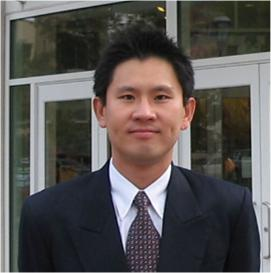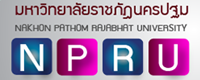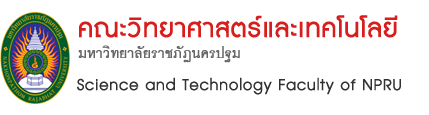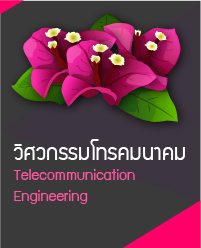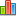Statistics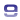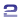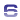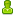User Online 1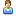Today 15Yesterday 3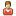This Month 24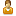Last Month 331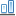This Year 2,451Last Year 4,261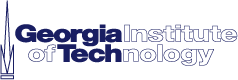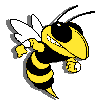Ph.D. Program (Electrical Engineering) ACADEMIC COURSEWORKS (Jan'00 - Present) 1. Advanced Digital Signal Processing (ECE 6250) An introduction to advanced signal processing methods that are used in a variety of applications areas. 2. Linear Systems and Controls (ECE 6550) Introduction to linear system theory and feedback control. Topics include state space representations, controllability and observability, linear feedback control. 3. Optimal Estimation (ECE 6555) Techniques for signal and state estimation in the presence of measurement and process noise with the emphasis on Wiener and Kalman filtering. 4. Advanced Digital Communications (ECE 6603) The theory and practice of efficient digital communications over linear dispersive channels, including adaptive equalization and synchronization. 5. Personal and Mobile Communications (ECE 6604) To introduce various topics that are fundamental to cellular mobile telephone systems. 6. Coding Theory and Applications (ECE 6606) To introduce the theory and practice of error control coding, with emphasis on linear, cyclic, convolutional, and parallel concatenated codes. 7. Research Seminar (ECE 8010) Seminar presentations describing Related research projects, centers, and other activities at Georgia Tech. 8. Doctoral Thesis (ECE 9000) N/A 9. Topics in Linear Algebra (MATH 4305)  Description Finite dimensional vector spaces, inner product spaces, least squares, linear transformations, the spectral theorem for normal transformations. Applications to convex sets, positive matrices, difference equations. 10. Algebra II (MATH 6122)  Description Graduate level linear and abstract algebra including rings, fields, modules, some algebraic number theory and Galois theory. (2nd of two courses) 11. Linear Statistical Models (MATH 6266) Basic unifying theory underlying techniques of regression, analysis of variance and covariance, from a geometric point of view. Modern computational capabilities are exploited fully. Students apply the theory to real data through canned and coded programs